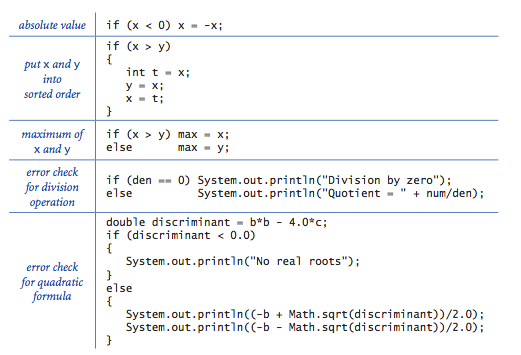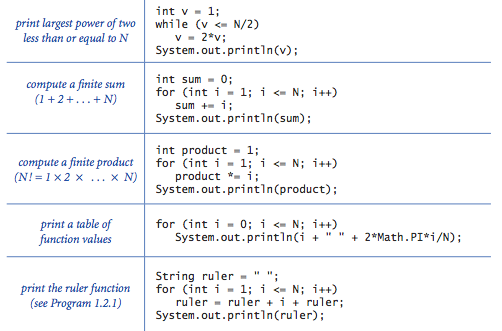# introduction to programming in java学习笔记(2)--循环

2015/11/11

## 1.3条件语句与循环

• if和if-else表达式
if (<boolean expression>) { <statements> }

• 布尔值来做判断是否执行后面的大括号内的内容
if (<boolean expression>) <statements T>
else <statements F>

• if 后面判断若为真就执行其后面语句,若为假则执行 else 后面的语句
• if和 if-else 图示• while循环
while (<boolean expression} { <statements> }

• 判断 while 后面()中的语句若为真则执行后面语句,循环执行直到为假结束
• while 图示• for循环
for (<initialize>; <boolean expression>; <increment>)
{ <statements> }

• 两个分号三个条件,第一个是初始化,第二个是判断语句,第三个循环控制条件
• 例如:一个简单的 loop 输出
for (int i = 4; i <= 10; i = i + 1)
System.out.println(i + "th Hello");

• 一个利用循环求解平方根的例子:
public class Sqrt {
public static void main(String[] args) {
double c = Double.parseDouble(args);
double epsilon = 1e-15;
double t = c;
while (Math.abs(t - c/t) > epsilon*t) {
t = (c/t + t) / 2.0;
}

System.out.println(t);
}

}

• 在 java中 库函数 Math.sqrt() ,就是用这种方法来求解的,这种方法的根本是 Newton’s method 的计算机语言实现,在这里可以看到很经典的两块内容,一个是控制循环条件中对精度的控制,没有达到精度就一直循环,函数体里面是对根t的求解方程,是由 Newton’s method 方法求解简单理解就是一个二次方程的根的求解,实质是 t2-c = 0 的求解根 t 的过程

• 其他的一些控制条件和循环
• break 语句跳出本次循环
• swith 语句选择语句
• do-while 循环
• 这些都是基本的循环语句也是一门语言必备的循环工具

#### 参考书籍

Introduction to Programming in Java

• 除非注明，本博文即为原创，转载请注明本博文链接地址
• 本博文只用于分享和交流知识，不得转载商用或个人牟利
• 如果您觉得文章对您有帮助，可以通过点击下面按钮分享